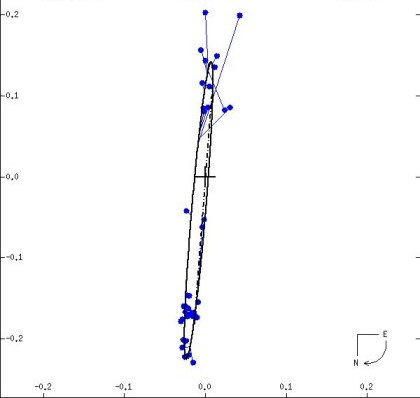THETA-1 TAU (Theta-1 Tauri). Right between Gamma Tauri (Hyadum I), within the Hyades Cluster at the point of the Taurus the Bull's head and Aldebaran (which is a foreground star), lies a seeming naked-eye binary, Theta Tauri (of no proper name), its two components separated by 337 seconds of arc (0.094 degree). The numerical suffixes on Theta-1 and Theta-2 have to do not with brightness, but with placement, Theta-1 to the west, following the usual convention. While fourth magnitude (3.84) Theta-1 is actually somewhat the lesser star (Theta-2 shining at third magnitude), it is special as one of the four evolved helium-fusing "K giants" of the Hyades, the others in brightness order being Epsilon Tau (Ain, with its planet, and actually classed G9.5), Gamma (Hyadum I), Delta-1 (Hyadum-II), and our star here, class K0 Theta-1. The star's measured distance of 154 light years (give or take 2) is right on the mark for the cluster's measured mean distance of 152 light years. Distance, a well-measured temperature of 4930 Kelvin (from which we can gauge the amount of infrared radiation), and a five percent allowance for light from a close companion (see below), yield a luminosity 66 times that of the Sun and a radius of 11.2 solar. Direct measure of angular size gives a satisfyingly close value of 11.7 times that of the Sun, showing the parameters to be close to correct. An equatorial rotation period of 3.9 kilometers per second give a rotation period of 144 days (0.39 years). If the rotational axis is tilted the same as the orbital axis of the companion (88 degrees to the line of sight), which seems likely, then that is also the true rotation period. Theory then gives us a mass of 2.5 times solar and an age of 640 million years, essentially the same as Theta-2 (670 million) and the Hyades in general (650 million), the agreement as it ought to be. Just a couple tenths of a second of arc away is a seventh magnitude companion, from its brightness probably an F8 dwarf with a temperature of around 6200 Kelvin, a luminosity of double that of the Sun, and a mass of 1.2 solar. Averaging 10.3 Astronomical Units from Theta-1 A, Theta-1 B takes 16.3 years to make a full orbit, a notable eccentricity taking it from 16 AU to 4.4 AU and back again.The companion to Theta-1 Tauri orbits its brighter mate (at the cross) every 16.3 years at a mean distance between 9.9 and 10.3 Astronomical Units. A severe eccentricity takes the two from as close as 4 AU to as far as 16. The orbital plane is nearly in the line of sight (the axis inclined by 88 degrees), which results in severe distortion of the actual ellipse and a large displacement of the actual major axis (dashed line). In reality, each orbits the other around a common center of mass. The scale on the axes is in tenths of a second of arc. Compare with Theta-2 Tauri's orbit. From the Sixth Catalog of Orbits of Visual Binary Stars , W. I. Hartkopf and B. D. Mason, US Naval Observatory Double Star Catalog, 2006.
Kepler's Laws give a total mass of 4.1 solar, more than the 3.7 solar derived from evolution and structure theory. If however, we reduce the mean separation to just 9.9 AU, not that much different, we can force agreement. Given the measured distances, Theta-1 and Theta-2 are nearly four light years apart, which would very much make it a non-double. However, given the errors of measurement, they could be at the same distance, making their minimum separation 16,000 AU, which given their masses would (assuming gravitational bonding) imply an orbital period of more than 700,000 years. Even then, however, gravitational perturbations from other cluster members would over time make such real duplicity unlikely at best. (Thanks to Paolo Colona, who suggested this star.)